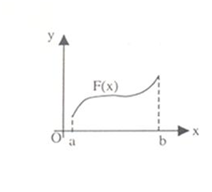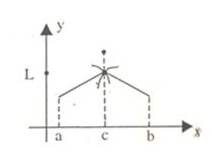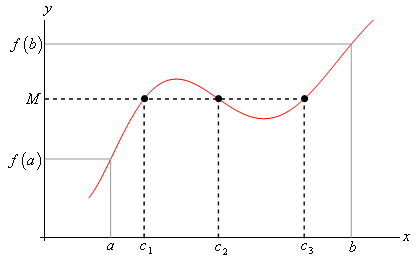# RD Sharma Class 12 and Chapter Continuity Maths Solutions

## Maths Class 12 and Chapter Continuity RD Sharma SolutionsFilter
Filters :
CLASSES
•
•
•
•
•
•
•
CHAPTERS
•
• 24 More

Preparing for exams is not an easy task as it's often said by the teachers and parents that timely practice and constant study can make you a better scorer in school. The truth is that no student can excel in class without having their concepts clear. Yes, you heard right. Be it any subject, a strong base with clear concepts are the core ingredients to make a happy recipe that you can taste lifelong.

Most of the students prefer RD Sharma when it comes to preparing thoroughly for board exams as it contains detailed chapters and easy-to-understand examples after every topic. Whether you believe it or not - the student who studies through these help guides is known to be better scorers than those who find NCERT enough to read and learn from.

I personally studied from RD Sharma, especially for maths, as NCERT has very limited questions and if you see board exam papers, they're full of tricky questions. The trickiness of these questions, however, increases in competitive exams like JEE Mains and BITS. But, thankfully, I had RD Sharma help guide for Maths and Physics which I practiced daily and tried to indulge concepts that are missing in NCERT books.

If you too genuinely want to score better and more than that want to have clear concepts and strong knowledge of maths tricks, then RD Sharma is the only savior you got till class 12th.

## INTRODUCTION: CONTINUITY

• A function f(x) is known to be continuous at x= a if

limx→a- f(x)= limx→a+ f(x)=f(a)

Thus, unlike limits, for continuity it is essential for the function to be defined at that point and the limiting value of the function should be equal to f(a).

• The function f(x) will be known to discontinuous at x =a in either of the following situations:

1. limx→a- f(x) and limx→a+ f(x) exist but are not equal.

2. limx→a- f(x) and limx→a+ f(x) exist and are equal but not equal to f(a).

3. f(a) is not defined.

4. At least one of the limits does not exist.

### IMPORTANT PROPERTIES OF CONTINUOUS FUNCTIONS:

If the functions f(x) and g(x) are both continuous at x =a then the following results hold true:

1. cf (x) is continuous at x =a where c is any constant.

2. f(x) + g(x) is continuous at x = a.

3. f(x).g(x) is continuous at x= a.

4. f(x)/g(x) is continuous at x= a, provided g(a) ≠ 0.

• A function is continuous at every point of (a, b), If a function f is continuous in (a, b).

• If f is continuous in [a, b] then in addition to being continuous at every point of the domain, f must also be continuous at the endpoints and f(x) is said to be continuous in the closed interval [a, b] if

1. f(x) will be continuous in (a, b).

2. limx→a+ f(x)=f(a)

limx→a- f(x)=f(a)• While solving problems on continuity, one need not calculate continuity at every point, in fact, the elementary knowledge of the function should be used to search the points of discontinuity.

• In questions like this where a function h is defined as

h(x) = f(x) for a < x < b

g(x) for b < x < c

The functions f and g are continuous in their respective intervals, then the continuity of function h should be checked only at the point x = b as this is the only possible point of discontinuity.

• If the point ‘a’ is finite, then the necessary and enough condition for the function f to be continuous at a is that limx→a- f(x) and limn→a+ f(x) should exist and be equal to f(a).

• A function continuous on a closed interval [a, b] is necessarily bounded if both a and b are finite. This is not valid in case of open interval.

• If the function u = f(x) is continuous at the point x=a, and the function y=g(u) is continuous at the point u = f(a), then the complex function y=(gof)(x)=g(f(x)) is continuous at the point x=a.

## DISCONTINUITY

If you know the graph of a function, it became easy to judge without even solving whether a function is continuous or not. The graph below clearly shows that the function is discontinuous.

• If limx→a- f(x) = L1 and limx→a+ f(x) = L2, where L1 and L2are both finite numbers then it is called ordinary discontinuity.• A function is said to have discontinuity of the second kind if neither limxf(x) nor lim- f(x) exists.

• If anyone of lim f(x) or limx f(x) exists and the other does not, then the function f is said to have a mixed discontinuity.

• If limf(x) exists but is not equal to f(a), then f(x) has removable discontinuity at x = a and it can be removed by redefining f(x) at x=a.

• If lim f(x) does not exist, then we can remove this discontinuity so that it becomes a non-removable or essential discontinuity.

• A function f(x) is said to have a jump discontinuity at a point x= a if, limx-f(x) ≠ limxf(x) and f(x) and may be equal to either of previous limits.

The concepts of limit and continuity are closely related to each other. If a function is continuous or not can be easily determined by the limit of the function.

## Intermediate Value Theorem

Suppose that f(x)f(x) is continuous on [a,b][a,b] and let MM be any number between f(a)f(a) and f(b)f(b). Then there exists a number cc such that,

1. a<c<ba<c<b

2. f(c)=Mf(c)=M

All the Intermediate Value Theorem is really saying is that a continuous function will take on all values between f(a)f(a) and f(b)f(b). Below is a graph of a continuous function that illustrates the Intermediate Value Theorem.As we can see from this image if we pick any value, MM, that is between the value of f(a)f(a) and the value of f(b)f(b) and draw a line straight out from this point the line will hit the graph in at least one point. In other words, somewhere between aa and bb the function will take on the value of MM. Also, as the figure shows the function may take on the value at more than one place.

It’s also important to note that the Intermediate Value Theorem only says that the function will take on the value of MM somewhere between aa and bb. It doesn’t say just what that value will be. It only says that it exists.

So, the Intermediate Value Theorem tells us that a function will take the value of MM somewhere between aa and bb but it doesn’t tell us where it will take the value nor does it tell us how many times it will take the value. These are important ideas to remember about the Intermediate Value Theorem.

## IMPORTANCE OF RD SHARMA CLASS 12TH Math

1. The 12th standard Mathematics RD Sharma provides students right concepts from the base and helps to understand the topics in a broad manner.

1. The candidates who constantly referred RD Sharma help guides for release the course to progress since it gives essential ideas instead of sounding the recipe-based inquiries straightforwardly.

1. We all realize that maths isn't writing type subject that we can learn Instead, it is a subject that requires opportune learning of recipes and rehearsing the inquiries as much as a candidate caliber permits.

1. RD Sharma twelfth class maths books have various practice questions and activities to measure to more readily understand the primary idea of inquiry and how to settle it.

1. Abstracted and gathered by training segment specialists, these books are organized according to the present test designs. The new release of the book contains all the ongoing changes and are twofold checked by experienced instructors.

1. As the book comprises of both, comprehended and unsolved inquiries, the candidates can discover the arrangements of such practice practices online also. So, RD Sharma books are assembled pleasantly and supported with online arrangements.